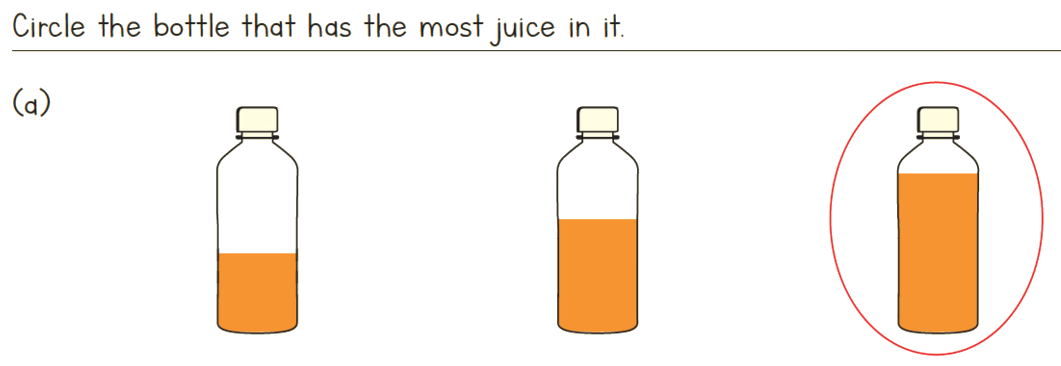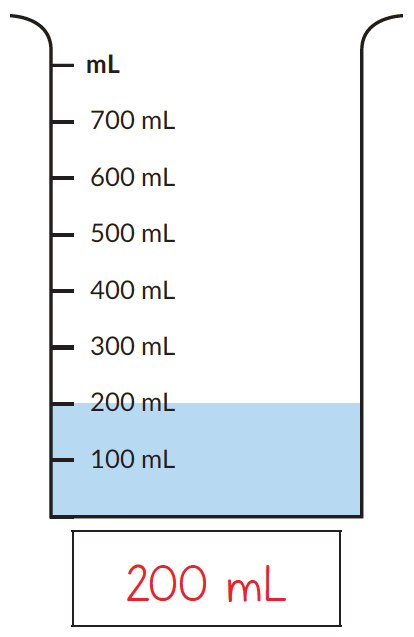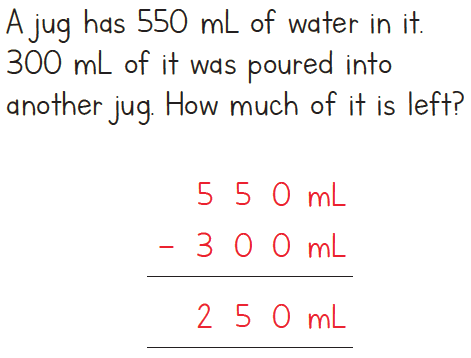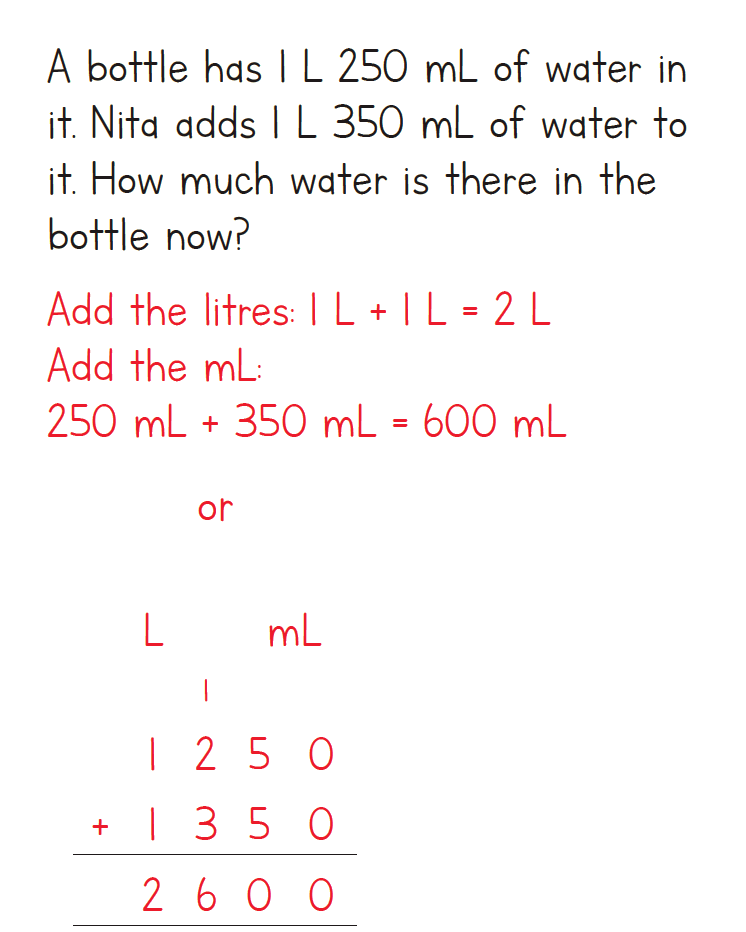# Measuring Capacity

Go back to  'Mensuration and Measurement'

## Measurement of capacity

Capacity is the maximum amount of liquid a container can hold. The concept of capacity begins by helping children understand it in simple terms like, empty, full, half full, half empty etc. An understanding of these concepts would set the stage for understanding the measurement of objects using standardised units.

## Non-standard units of measuring capacity

Non-standard units of measurement are used in early years of learning, to introduce children to the concept of measuring without them having to read any scales. Reading scales of any kind is a difficult skill in itself, so the idea of non-standard measures is to focus the child on the concept of empty, full, a little empty, before they move onto the next step of measuring using standard units.

To measure capacity, you can give daily life examples like, while cooking rice we add 1 or 2 cups of water. Explaining the child that this is a non-standard way of measuring capacity.## Standard units to measure capacity

Just like the way we have units to measure length and weight, we have standard units to measure capacity.  Children learn to use standard units of measurement in Grade 2, They learn about different units to measure capacity. They also learn about how to read scales on different instruments which measure capacity. Children also learn about the relationship between units of measurement. They would need to know the following fact:

### Formula

1000 millilitres (ml) = 1 litre (l)An example showing the capacity of the beaker.

Using this fact, they would be able to convert the capacities. Add and subtract capacities. A thorough understanding of the conversion of units can be built by exposing children to real life situations where measuring converting and figuring out the solution happens.Children would continue this kind of problem-solving in until Grade 5. They would solve application based problems involving all four operations (addition, subtraction, multiplication and division).

## Tips and tricks

• Remember that most medicine bottles come with the capacity of a few ml. They are small and generally have a capacity of 50 ml or 100 ml or 200 ml. On the other hand, a much larger-looking bottle is that of drinking water. This is most typically seen in 1-litre size. Using these commonly seen items to anchor the units helps children.
Measurement of Length, Weight, and Capacity
Measurement of Length, Weight, and Capacity
An Introduction to Measurement
An Introduction to Measurement
Measurement of weight and capacity